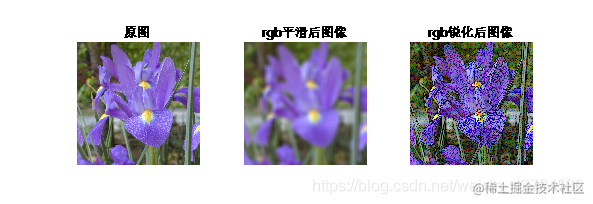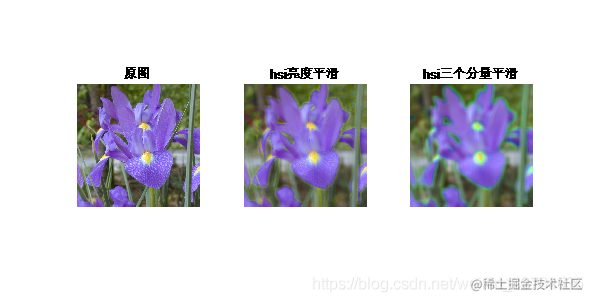# 数字图像处理Matlab-彩色图像处理（附代码）

## 1.Objectives:

1、学习 RGB、Indexed 和 Gray 图像间转换函数 2、掌握彩色图像平滑与锐化的算法 3、 学习彩色图像的分割方法

## 3.Experiment Principle:

（冈萨雷斯著作，电子工业出版社于2009年12月1日出版）

## 4.Experiment Steps Result and Conlusion：

### 2、彩色图像平滑锐化第一张图进行滤波就是在rgb上滤波，看到整个图像变得平滑，比较模糊，右上图整个图像只要有颜色变化的地方都变得尖锐起来，能看到明显的颜色变化，在hsi空间上的滤波，看到旨在i强度上平滑的效果，使得各个颜色的强度变化不大，就是每种颜色都比较突出，hsi所有的进行滤波，发现整个图就看不太清楚。

## 【附录】实现代码

``````clear all;clc;
[f1,map1]=rgb2ind(f,8,'nodither');%将rgb图像转化为索引图像，且索引图像为8种颜色，不抖动
figure(1);
subplot(1,2,1);
imshow(f1,map1);
title("rgb2ind无抖动");
[f2,map2]=rgb2ind(f,8,'dither');%将rgb图像转化为索引图像，且索引图像为8种颜色，有抖动
subplot(1,2,2);
imshow(f2,map2);
title("rgb2indy有抖动");

f3=rgb2gray(f);%将rgb图像转化为灰度图像，且灰度图像为8种颜色，不抖动
figure(2);
subplot(1,2,1);
imshow(f3,[]);
title("rgb2gray无抖动");
f4=dither(f3);
subplot(1,2,2);imshow(f4,[]);
title("rgb2gray有抖动");

``````clear all;clc;
w=ones(25)./(25*25);
f_filtered=imfilter(f,w,'replicate');
figure(1);
subplot(1,3,1);
imshow(f,[]);
title("原图");
subplot(1,3,2);
imshow(f_filtered,[]);
title("rgb平滑后图像");
fb=f;w=[1 1 1 1 1;1 1 1 1 1;1 1 -24 1 1;1 1 1 1 1;1 1 1 1 1];%拉普拉斯算子
fen=fb-imfilter(fb,w,'replicate');%锐化处理
subplot(1,3,3);imshow(fen,[]);title("rgb锐化后图像");

hsi=rgb2hsi(f);%得到hsi空间的图像
h=hsi(:,:,1);%得到hsi空间的色调，饱和度，亮度分量
s=hsi(:,:,2);i=hsi(:,:,3);
w=ones(25)./(25*25);%滤波器参数
i_filtered=imfilter(i,w,'replicate');%对亮度进行平滑处理
hsi1=cat(3,h,s,i_filtered);
f1=hsi2rgb(hsi1);%转化为rgb图像
figure(2);
subplot(1,3,1);imshow(f,[]);title("原图");
subplot(1,3,2);imshow(f1,[]);title("hsi亮度平滑");

h_filtered=imfilter(h,w,'replicate');
i_filtered=imfilter(i,w,'replicate');
s_filtered=imfilter(s,w,'replicate');
hsi2=cat(3,h_filtered,s_filtered,i_filtered);
f2=hsi2rgb(hsi2);%转化为rgb图像
subplot(1,3,3);imshow(f2,[]);title("hsi三个分量平滑");

``````clear all;clc;
figure(2);
imshow(rgb_image);
g=cat(3,red,green,blue);
figure(3);
imshow(g);
%显示感兴趣区域

%求感兴趣区域的均值与协方差矩阵
[M,N,K]=size(g);
I=reshape(g,M*N,3);%将g变成一个m*n行3列的矩阵
I=double(I(idx,1:3));
[C,m]=covmatrix(I);%求协矩阵与均值
d=diag(C);%返回矩阵对角线行的元素
sd=sqrt(d);%求出标准差

E25=colorseg('euclidean',rgb_image,25,m);%这里使用'euclidean'进行分割，选取阈值为25
M25=colorseg('mahalanobis',rgb_image,25,m,C);%这里使用'mahalanobis'进行分割，选取阈值为25
figure(4);
subplot(211);imshow(E25);
subplot(212);imshow(M25);